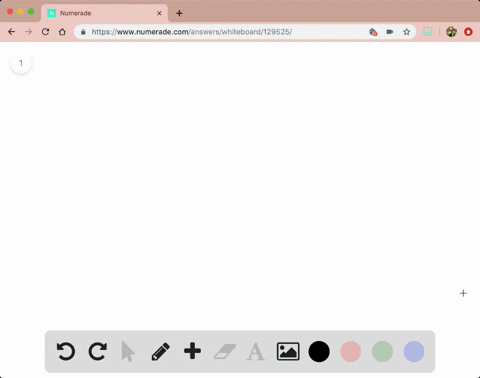Enroll in one of our FREE online STEM bootcamps. Join today and start acing your classes!View Bootcamps### Problem 10

03:27University of California, Riverside
Problem 9

# Answer the following questions about the functions whose derivatives are given:\begin{equation}\begin{array}{l}{\text { a. What are the critical points of } f ?} \\ {\text { b. On what open intervals is } f \text { increasing or decreasing? }} \\ {\text { c. At what points, if any, does } f \text { assume local maximum and }} \\ \quad {\text { minimum values? }}\end{array}\end{equation}\begin{equation}f^{\prime}(x)=1-\frac{4}{x^{2}}, \quad x \neq 0\end{equation}

## Discussion

You must be signed in to discuss.

## Video Transcript

Okay, So for prom night, um, if the ribs were given ISS one minus four over X squared where asked Ex cannot be zero. So the first thing we need to do is to find the critical points that is to let the derivative to be equal to zero on DDE This case, we can find a solution, which is positive or negative too. Okay, now for party, let's first let the derivative to be positive now, by solving this inequality we can find. So the first thing we can do is to won't apply X squared on both sides. So that's X squared minus four, bigger than zero. So that means X squared is strictly bigger dead for hens. Our acts should be bigger than two or X. Be smaller than next to. So that means, um, like to be ability to thank you too. Union Two, two You been t on this interval function will be increasing and I think to to to his function will be decreasing. Oh, I'm sorry. Um, remember that we don't allow X to be zero, so we have to act. I have to exclude zero from this interval, so that means our our interval for decreasing will be from negative 2 to 0. Union 02 hostile to. Okay, now for Parsi. Now. See you. We need Thio. We need to find a local Mexico. My local minimum. Well, in this case, we observed these two intervals. Then we can find, uh, connected to we'll be the local maximum and half of two will be the local minimum.